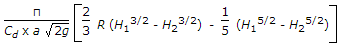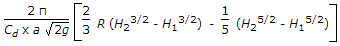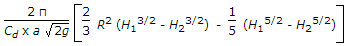# Mechanical Engineering - Hydraulics and Fluid Mechanics

### Exercise :: Hydraulics and Fluid Mechanics - Section 6

6.

The coefficient of venturifiume, generally, lies between

 A. 0.3 to 0.45 B. 0.50 to 0.75 C. 0.75 to 0.95 D. 0.95 to 1.0

Answer: Option D

Explanation:

No answer description available for this question. Let us discuss.

7.

Venturimeter is used to

 A. measure the velocity of a flowing liquid B. measure the pressure of a flowing liquid C. measure the discharge of liquid flowing in a pipe D. measure the pressure difference of liquid flowing between two points in a pipe line

Answer: Option C

Explanation:

No answer description available for this question. Let us discuss.

8.

A hemispherical tank of radius (R) containing liquid upto height (H1) has an orifice of cross-sectional area (a) at its bottom. The time required to lower the level of liquid from (H1) to (H2) will be

 A.B.C.D. none of the above

Answer: Option C

Explanation:

No answer description available for this question. Let us discuss.

9.

A flow is called super-sonic if the

 A. velocity of flow is very high B. discharge is difficult to measure C. Mach number is between 1and 6 D. none of these

Answer: Option C

Explanation:

No answer description available for this question. Let us discuss.

10.

The force present in a moving liquid is

 A. inertia force B. viscous force C. gravity force D. all of these

Answer: Option D

Explanation:

No answer description available for this question. Let us discuss.

#### Current Affairs 2021

Interview Questions and Answers